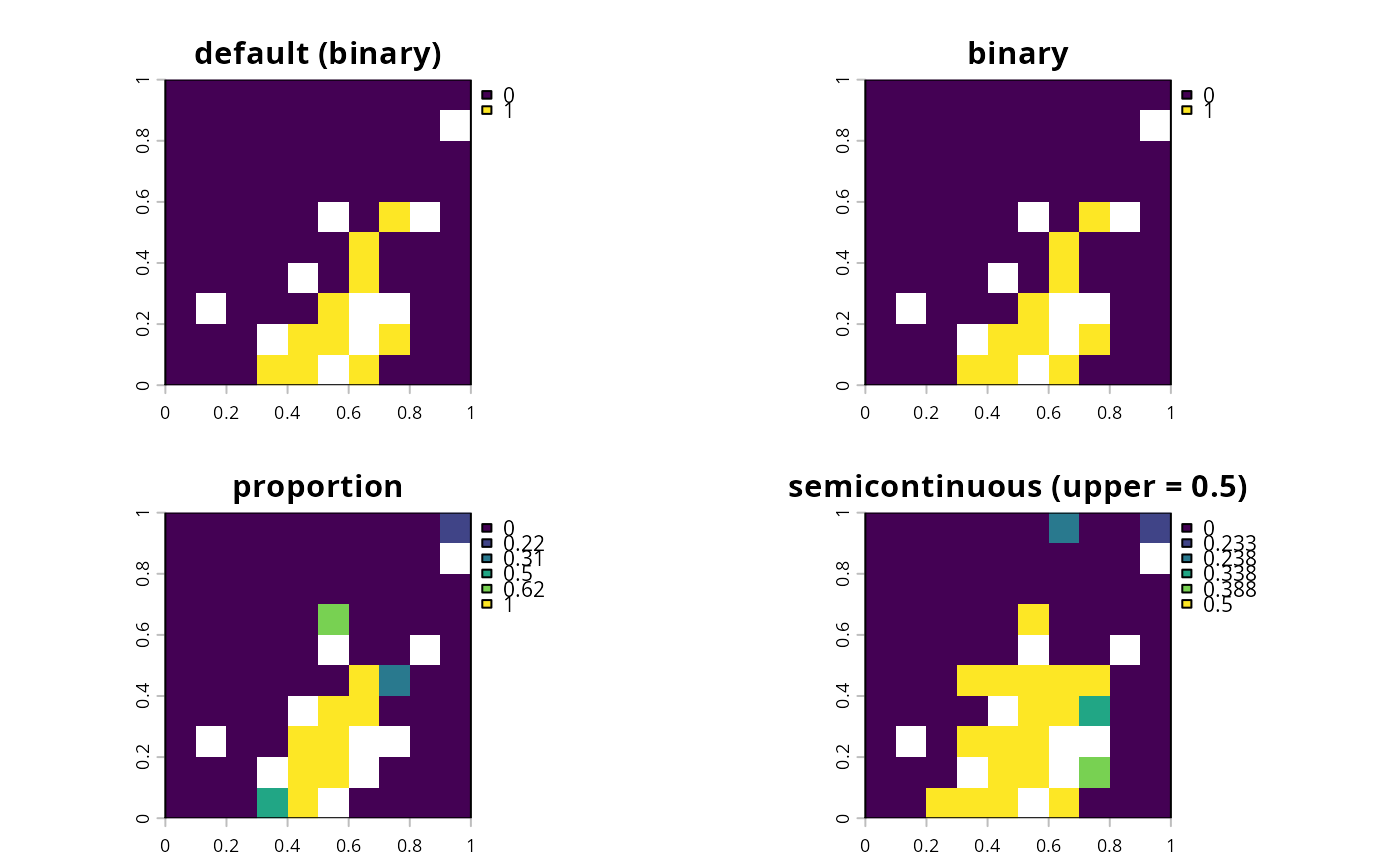Conservation planning problems involve making decisions on how different planning units will be managed. These decisions might involve turning an entire planning unit into a protected area, turning part of a planning unit into a protected area, or allocating a planning unit to a specific management zone. If no decision is explicitly added to a problem, then binary decisions will be used by default.

## Details

Only a single type of decision can be added to a conservation planning problem(). Note that if multiple decisions are added to a problem, then the last one added will be used.

The following decisions can be added to a conservation planning problem():

add_binary_decisions()

Add a binary decision to a conservation planning problem. This is the classic decision of either prioritizing or not prioritizing a planning unit. Typically, this decision has the assumed action of buying the planning unit to include in a protected area network. If no decision is added to a problem object then this decision class will be used by default.

add_proportion_decisions()

Add a proportion decision to a conservation planning problem. This is a relaxed decision where a part of a planning unit can be prioritized, as opposed to the default of the entire planning unit. Typically, this decision has the assumed action of buying a fraction of a planning unit to include in a protected area network.

add_semicontinuous_decisions()

Add a semi-continuous decision to a conservation planning problem. This decision is similar to add_proportion_decision except that it has an upper bound parameter. By default, the decision can range from prioritizing none (0%) to all (100%) of a planning unit. However, a upper bound can be specified to ensure that at most only a fraction (e.g., 80%) of a planning unit can be preserved. This type of decision may be useful when it is not practical to conserve the entire area encompassed by any single planning unit.

Other overviews: constraints, importance, objectives, penalties, portfolios, solvers, summaries, targets

## Examples

# \dontrun{
sim_pu_raster <- get_sim_pu_raster()
sim_features <- get_sim_features()

# create basic problem and using the default decision types (binary)
p1 <-
problem(sim_pu_raster, sim_features) %>%

# create problem with manually specified binary decisions

# create problem with proportion decisions

# create problem with semicontinuous decisions
p4 <- p1 %>% add_semicontinuous_decisions(upper_limit = 0.5)

# solve problem
s <- c(solve(p1), solve(p2), solve(p3), solve(p4))
names(s) <- c(
"default (binary)", "binary", "proportion", "semicontinuous (upper = 0.5)"
)
# plot solutions
plot(s)# }